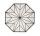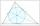# Right triangle - math word problems

1. Cone containerRotary cone-shaped container has a volume 1000 cubic cm and a height 12 cm. Calculate how much metal we need for making this package.
2. Octagonal matOctagonal mat formed from a square plate with a side of 40 cm so that every corner cut the isosceles triangle with leg 3.6 cm. What is the content area of one mat?
3. Lamp coneCalculate the surface of a lamp shade shaped of a rotary truncated cone with base diameter 32 cm and 12 cm and height 24 cm.
4. CloudsFrom two points A and B on the horizontal plane was observed forehead cloud above the two points under elevation angle 73°20' and 64°40'. Points A , B are separated by 2830 m. How high is the cloud?
5. Is right triangleOne angle of the triangle is 36° and the remaining two are in the ratio 3:5. Determine whether triangle is a rectangular triangle.
6. PrismThe base of a perpendicular triangular prism is a right triangle with legs 4.5 cm and 6 cm long. What is the surface of the prism, if its volume is 54 cubic centimeters?
7. Perimeter of circleCalculate the circumference of described circle to the triangle with sides 9,12,15 cm.
8. Triangular prismBase of perpendicular triangular prism is a right triangle with leg length 5 cm. Content area of the largest side wall of its surface is 130 cm² and the height of the body is 10 cm. Calculate its volume.
9. Triangle KLBIt is given equilateral triangle ABC. From point L which is the midpoint of the side BC of the triangle it is drwn perpendicular to the side AB. Intersection of perpendicular and the side AB is point K. How many % of the area of the triangle ABC is area o
10. Hexa prismDetermine the volume of hex prism with edge base 4 cm. The body height is 28 cm.
11. Vertical prismThe base of vertical prism is a right triangle with leg a = 5 cm and a hypotenuse c = 13 cm. The height of the prism is equal to the circumference of the base. Calculate the surface area and volume of the prism
12. Two ballsTwo balls, one 8cm in radius and the other 6cm in radius, are placed in a cylindrical plastic container 10cm in radius. Find the volume of water necessary to cover them.
13. Diagonals in diamons/rhombusRhombus ABCD has side length AB = 4 cm and a length of one diagonal of 6.4 cm. Calculate the length of the other diagonal.
14. The ditchDitch with cross section of an isosceles trapezoid with bases 2m 6m are deep 1.5m. How long is the slope of the ditch?
15. 6 regular polygonIt is given 6 side regular polygon whose side is 5 cm. Calculate its content area. Compare how many more cm2 (square centimeters) has a circle in which is inscribed the 6-gon.
16. Complete constructionConstruct triangle ABC if hypotenuse c = 7 cm and angle ABC = 30 degrees. / Use Thales' theorem - circle /. Measure and write down the length of legs.
17. Inscribed circleWrite the equation of a incircle of the triangle KLM if K [2,1], L [6,4], M [6,1].
18. RhombusABCD is a rhombus, ABD is an equilateral triangle and AC is equal to 4. Find the area of the rhombus.On a straight stretch of road is marked 12 percent drop. What angle makes the direction of the road with the horizontal plane?
20. Hexagon areaThe center of the regular hexagon is 21 cm away from its side. Calculate the hexagon side and its area.

Do you have an interesting mathematical word problem that you can't solve it? Enter it, and we can try to solve it.

We will send a solution to your e-mail address. Solved examples are also published here. Please enter the e-mail correctly and check whether you don't have a full mailbox.

Please do not submit problems from current active competitions such as Mathematical Olympiad, correspondence seminars etc...